## Saturday, 9 August 2014

### chapter 12 Worked Out Examples 3

 Example: 5
Evaluate the following limits:
 (a)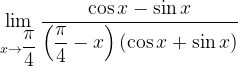$\mathop {\lim }\limits_{x \to \dfrac{\pi }{4}} \dfrac{{\cos x - \sin x}}{{\left( {\dfrac{\pi }{4} - x} \right)\left( {\cos x + \sin x} \right)}}$ (b)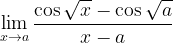$\mathop {\lim }\limits_{x \to a} \dfrac{{\cos \sqrt x - \cos \sqrt a }}{{x - a}}$ (c)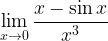$\mathop {\lim }\limits_{x \to 0} \dfrac{{x - \sin x}}{{{x^3}}}$ (d)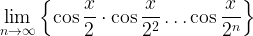$\mathop {\lim }\limits_{n \to \infty } \left\{ {\cos \dfrac{x}{2} \cdot \cos \dfrac{x}{{{2^2}}}\ldots\cos \dfrac{x}{{{2^n}}}} \right\}$
 Solution: 5-(a)
Before proceeding to solve these limits, it should be mentioned that most limits can be evaluated in more than one manner. (In fact, L’Hospital’s rule is a technique that can be used to solve most indeterminate limits: we will discuss it in detail in the topic of differentiation). In these examples and the ones that follow we will be discussing multiple ways for solving limits, wherever they are important.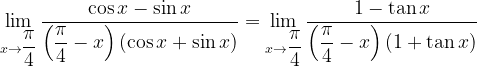$\mathop {\lim }\limits_{x \to \dfrac{\pi }{4}} \dfrac{{\cos x - \sin x}}{{\left( {\dfrac{\pi }{4} - x} \right)\left( {\cos x + \sin x} \right)}} = \mathop {\lim }\limits_{x \to \dfrac{\pi }{4}} \dfrac{{1 - \tan x}}{{\left( {\dfrac{\pi }{4} - x} \right)\left( {1 + \tan x} \right)}}$ (Dividing Num and Den by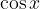$\cos x$)
Let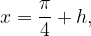$x = \dfrac{\pi }{4} + h,$ so that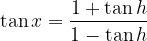$\tan x = \dfrac{{1 + \tan h}}{{1 - \tan h}}$ and the limit above becomes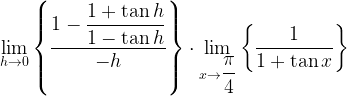$\mathop {\lim }\limits_{h \to 0} \left\{ {\dfrac{{1 - \dfrac{{1 + \tan h}}{{1 - \tan h}}}}{{ - h}}} \right\} \cdot \mathop {\lim }\limits_{x \to \dfrac{\pi }{4}} \left\{ {\dfrac{1}{{1 + \tan x}}} \right\}$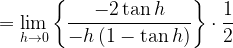$= \mathop {\lim }\limits_{h \to 0} \left\{ {\dfrac{{ - 2\tan h}}{{ - h\left( {1 - \tan h} \right)}}} \right\} \cdot \dfrac{1}{2}$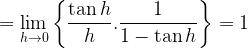$= \mathop {\lim }\limits_{h \to 0} \left\{ {\dfrac{{\tan h}}{h}.\dfrac{1}{{1 - \tan h}}} \right\} = 1$
Alternatively, we could proceed by multiplying the numerator and denominator of the original limit by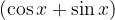$(\cos x + \sin x)$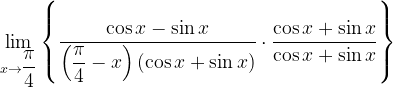$\mathop {\lim }\limits_{x \to \dfrac{\pi }{4}} \left\{ {\dfrac{{\cos x - \sin x}}{{\left( {\dfrac{\pi }{4} - x} \right)\left( {\cos x + \sin x} \right)}} \cdot \dfrac{{\cos x + \sin x}}{{\cos x + \sin x}}} \right\}$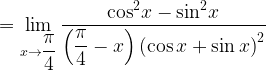$= \mathop {\lim }\limits_{x \to \dfrac{\pi }{4}} \dfrac{{{{\cos }^2}x - {{\sin }^2}x}}{{\left( {\dfrac{\pi }{4} - x} \right){{\left( {\cos x + \sin x} \right)}^2}}}$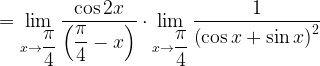$= \mathop {\lim }\limits_{x \to \dfrac{\pi }{4}} \dfrac{{\cos 2x}}{{\left( {\dfrac{\pi }{4} - x} \right)}}\cdot \mathop {\lim }\limits_{x \to \dfrac{\pi }{4}} \dfrac{1}{{{{\left( {\cos x + \sin x} \right)}^2}}}$$= \mathop {\lim }\limits_{h \to 0} \dfrac{{ - \sin 2h}}{{ - h}} \cdot \dfrac{1}{2}\left( {{\rm{we\, let\, }}x = \dfrac{\pi }{4} + h{\rm{so \,that}}\,\cos 2x = \cos \left( {\dfrac{\pi }{2} + 2h} \right) = - \sin 2h} \right)$$= \mathop {\lim }\limits_{h \to 0} \dfrac{{\sin 2h}}{{2h}}$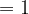$=1$
 Solution: 5-(b)$\mathop {\lim }\limits_{x \to a} \dfrac{{\cos \sqrt x - \cos \sqrt a }}{{x - a}}$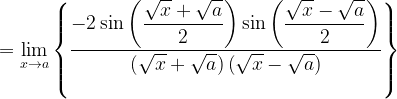$= \mathop {\lim }\limits_{x \to a} \left\{ {\dfrac{{ - 2\sin \left( {\dfrac{{\sqrt x + \sqrt a }}{2}} \right)\sin \left( {\dfrac{{\sqrt x - \sqrt a }}{2}} \right)}}{{\left( {\sqrt x + \sqrt a } \right)\left( {\sqrt x - \sqrt a } \right)}}} \right\}$
{We used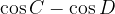$\cos C - \cos D$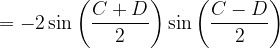$= - 2\sin \left( {\dfrac{{C + D}}{2}} \right)\sin \left( {\dfrac{{C - D}}{2}} \right)$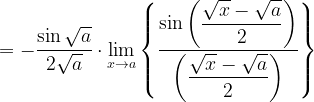$= - \dfrac{{\sin \sqrt a }}{{2\sqrt a }} \cdot \mathop {\lim }\limits_{x \to a} \left\{ {\dfrac{{\sin \left( {\dfrac{{\sqrt x - \sqrt a }}{2}} \right)}}{{\left( {\dfrac{{\sqrt x - \sqrt a }}{2}} \right)}}} \right\}$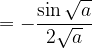$= - \dfrac{{\sin \sqrt a }}{{2\sqrt a }}$
 Solution: 5-(c)
This limit can be solved very easily by using the expansion series for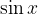$\sin x$: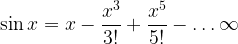$\sin x = x - \dfrac{{{x^3}}}{{3!}} + \dfrac{{{x^5}}}{{5!}} - \ldots\infty$
Therefore,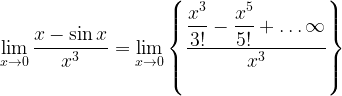$\mathop {\lim }\limits_{x \to 0} \dfrac{{x - \sin x}}{{{x^3}}} = \mathop {\lim }\limits_{x \to 0} \left\{ {\dfrac{{\dfrac{{{x^3}}}{{3!}} - \dfrac{{{x^5}}}{{5!}} + \ldots\infty }}{{{x^3}}}} \right\}$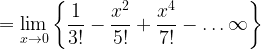$= \mathop {\lim }\limits_{x \to 0} \left\{ {\dfrac{1}{{3!}} - \dfrac{{{x^2}}}{{5!}} + \dfrac{{{x^4}}}{{7!}} - \ldots\infty } \right\}$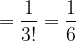$= \dfrac{1}{{3!}} = \dfrac{1}{6}$
Alternatively, we know that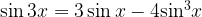$\sin 3x = 3\sin x - 4{\sin ^3}x$
Hence,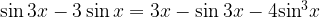$\sin 3x - 3\sin x = 3x - \sin 3x - 4{\sin ^3}x$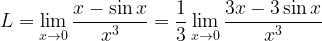$L = \mathop {\lim }\limits_{x \to 0} \dfrac{{x - \sin x}}{{{x^3}}} = \dfrac{1}{3}\mathop {\lim }\limits_{x \to 0} \dfrac{{3x - 3\sin x}}{{{x^3}}}$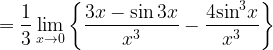$= \dfrac{1}{3}\mathop {\lim }\limits_{x \to 0} \left\{ {\dfrac{{3x - \sin 3x}}{{{x^3}}} - \dfrac{{4{{\sin }^3}x}}{{{x^3}}}} \right\}$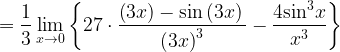$= \dfrac{1}{3}\mathop {\lim }\limits_{x \to 0} \left\{ {27 \cdot \dfrac{{\left( {3x} \right) - \sin \left( {3x} \right)\,}}{{{{\left( {3x} \right)}^3}}} - \dfrac{{4{{\sin }^3}x}}{{{x^3}}}} \right\}$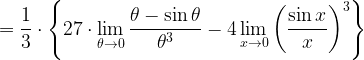$= \dfrac{1}{3} \cdot \left\{ {27 \cdot \mathop {\lim }\limits_{\theta \to 0} \dfrac{{\theta - \sin \theta }}{{{\theta ^3}}} - 4\mathop {\lim }\limits_{x \to 0} {{\left( {\dfrac{{\sin x}}{x}} \right)}^3}} \right\}$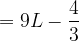$= 9L - \dfrac{4}{3}$
Hence,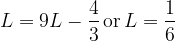$L = 9L - \dfrac{4}{3}\,{\rm{or}}\,L = \dfrac{1}{6}$
 Solution: 5-(d)
As in Example –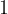$1$ Part – (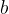$b$), we try to reduce this expression into a closed form by multiplying it with an appropriate factor as follows:

Now, as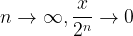$n \to \infty ,\dfrac{x}{{{2^n}}} \to 0$ and hence$\mathop {\lim }\limits_{n \to \infty } \left\{ {\cos \dfrac{x}{2} \cdot \cos \dfrac{x}{{{2^2}}} \ldots\cos \dfrac{x}{{{2^n}}} \cdot \dfrac{{\sin \dfrac{x}{{{2^n}}}}}{{\sin \dfrac{x}{{2n}}}}} \right\}$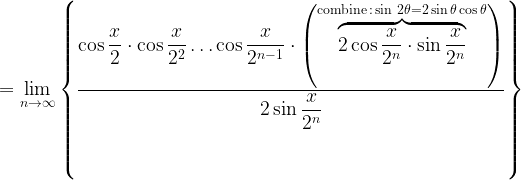$= \mathop {\lim }\limits_{n \to \infty } \left\{ {\dfrac{{\cos \dfrac{x}{2} \cdot \cos \dfrac{x}{{{2^2}}}\ldots\cos \dfrac{x}{{{2^{n - 1}}}} \cdot \left( {\overbrace {2\cos \dfrac{x}{{{2^n}}} \cdot \sin \dfrac{x}{{{2^n}}}}^{{\rm{combine}}\,:\,\sin \,2\theta = 2\sin \theta \cos \theta }} \right)}}{{2\sin \dfrac{x}{{{2^n}}}}}} \right\}$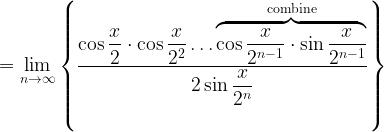$= \mathop {\lim }\limits_{n \to \infty } \left\{ {\dfrac{{\cos \dfrac{x}{2} \cdot \cos \dfrac{x}{{{2^2}}}\ldots\overbrace {\cos \dfrac{x}{{{2^{n - 1}}}} \cdot \sin \dfrac{x}{{{2^{n - 1}}}}}^{{\rm{combine}}}}}{{2\sin \dfrac{x}{{{2^n}}}}}} \right\}$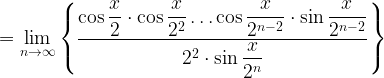$= \mathop {\lim }\limits_{n \to \infty } \left\{ {\dfrac{{\cos \dfrac{x}{2} \cdot \cos \dfrac{x}{{{2^2}}}\ldots\cos \dfrac{x}{{{2^{n - 2}}}} \cdot \sin \dfrac{x}{{{2^{n - 2}}}}}}{{{2^2} \cdot \sin \dfrac{x}{{{2^n}}}}}} \right\}$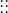$\vdots \vdots$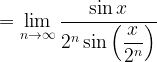$= \mathop {\lim }\limits_{n \to \infty } \dfrac{{\sin x}}{{{2^n}\sin \left( {\dfrac{x}{{{2^n}}}} \right)}}$$= \mathop {\lim }\limits_{n \to \infty } \dfrac{{\sin x}}{{\dfrac{{x \cdot \left( {\sin \left( {\dfrac{x}{{{2^n}}}} \right)} \right)}}{{\left( {\dfrac{x}{{{2^n}}}} \right)}}}} = \dfrac{{\sin x}}{x} \cdot \dfrac{1}{{\mathop {\lim }\limits_{n \to \infty } \left\{ {\dfrac{{\sin \left( {\dfrac{x}{{{2^n}}}} \right)}}{{\left( {\dfrac{x}{{{2^n}}}} \right)}}} \right\}}}$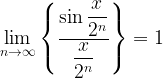$\mathop {\lim }\limits_{n \to \infty } \left\{ {\dfrac{{\sin \dfrac{x}{{{2^n}}}}}{{\dfrac{x}{{{2^n}}}}}} \right\} = 1$
Therefore, our final result is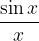$\dfrac{{\sin x}}{x}$